Скачать презентацию AGENDA Why has the mathematics program changed

c9d97e96ee3ed628ab40ae46c85110ac.ppt

• Количество слайдов: 46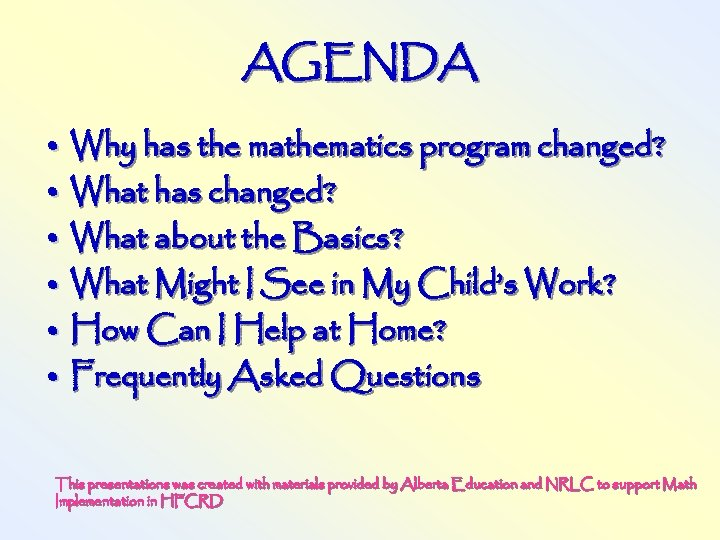AGENDA • Why has the mathematics program changed? • What has changed? • What about the Basics? • What Might I See in My Child’s Work? • How Can I Help at Home? • Frequently Asked Questions This presentations was created with materials provided by Alberta Education and NRLC to support Math Implementation in HFCRD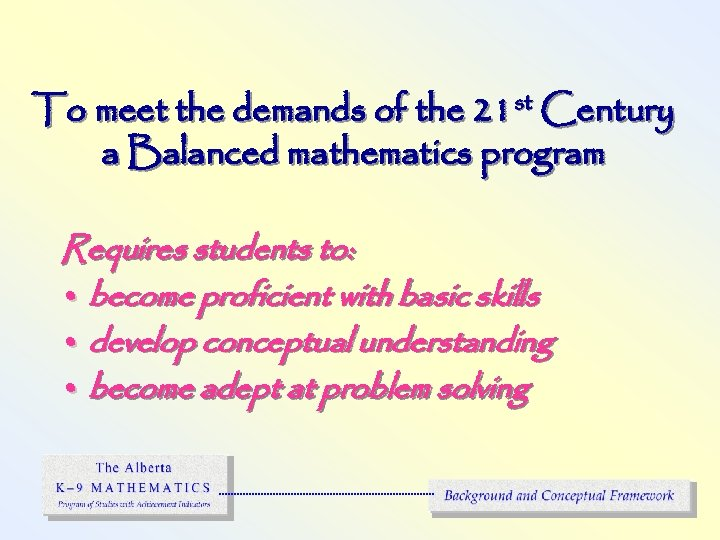To meet the demands of the 21 st Century a Balanced mathematics program Requires students to: • become proficient with basic skills • develop conceptual understanding • become adept at problem solving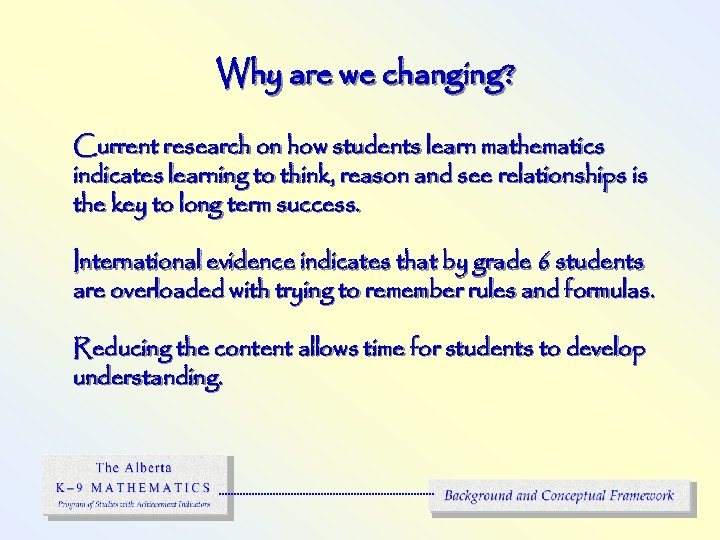Why are we changing? Current research on how students learn mathematics indicates learning to think, reason and see relationships is the key to long term success. International evidence indicates that by grade 6 students are overloaded with trying to remember rules and formulas. Reducing the content allows time for students to develop understanding.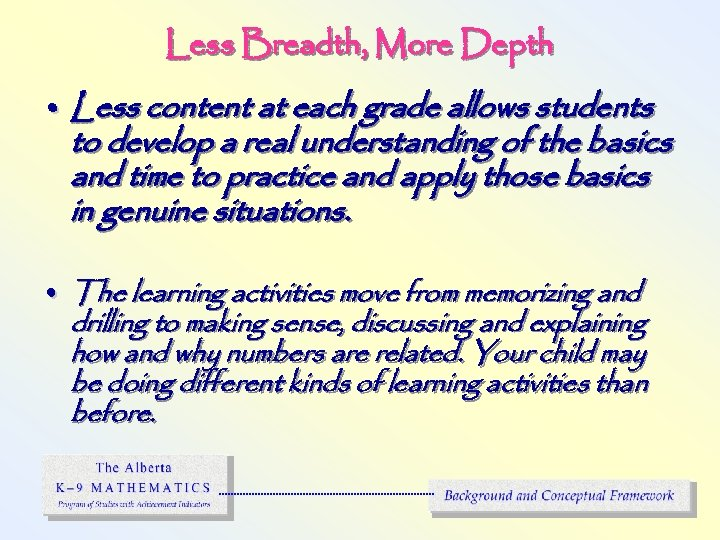Less Breadth, More Depth • Less content at each grade allows students to develop a real understanding of the basics and time to practice and apply those basics in genuine situations. • The learning activities move from memorizing and drilling to making sense, discussing and explaining how and why numbers are related. Your child may be doing different kinds of learning activities than before.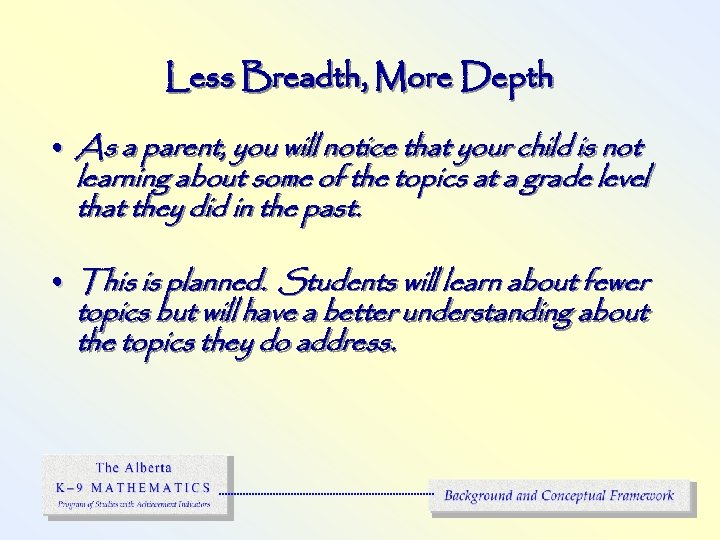Less Breadth, More Depth • As a parent, you will notice that your child is not learning about some of the topics at a grade level that they did in the past. • This is planned. Students will learn about fewer topics but will have a better understanding about the topics they do address.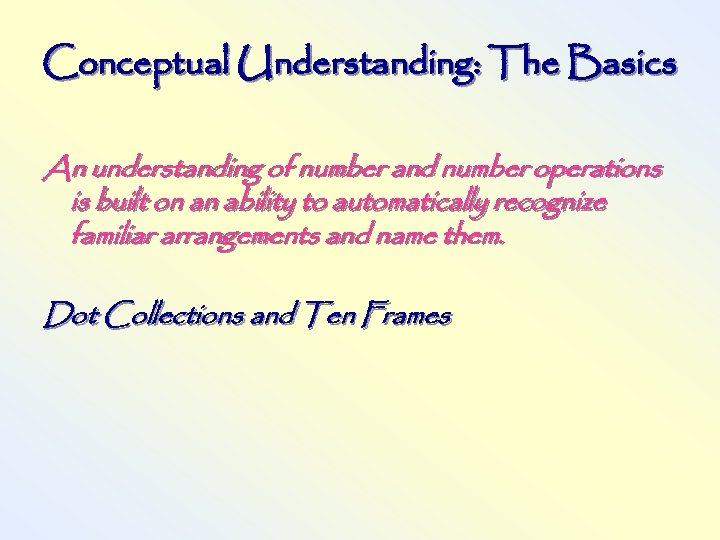Conceptual Understanding: The Basics An understanding of number and number operations is built on an ability to automatically recognize familiar arrangements and name them. Dot Collections and Ten Frames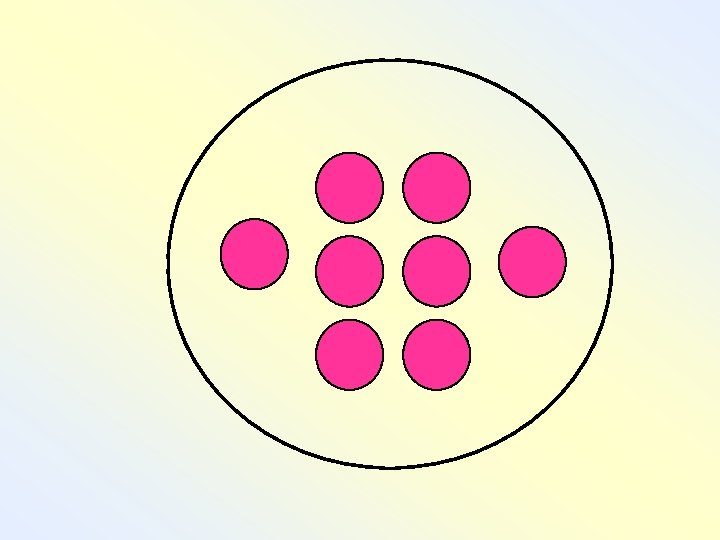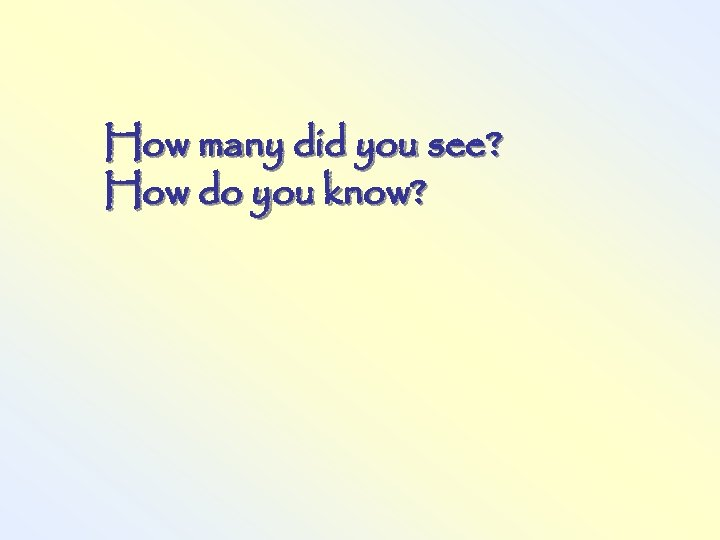How many did you see? How do you know?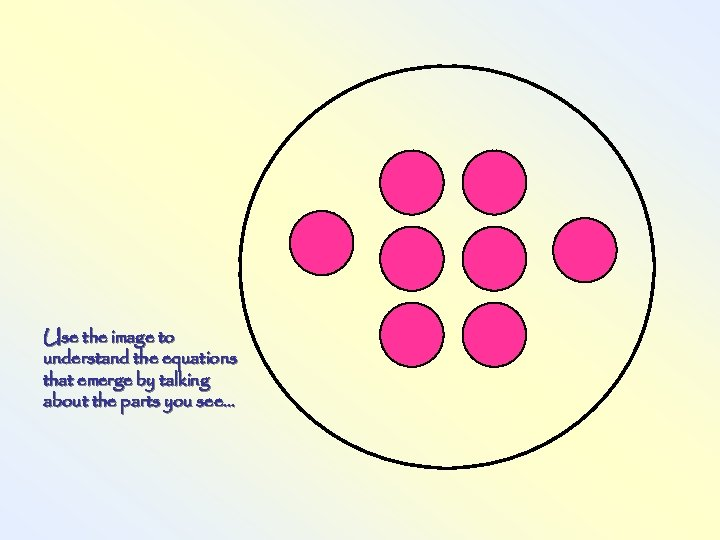Use the image to understand the equations that emerge by talking about the parts you see. . .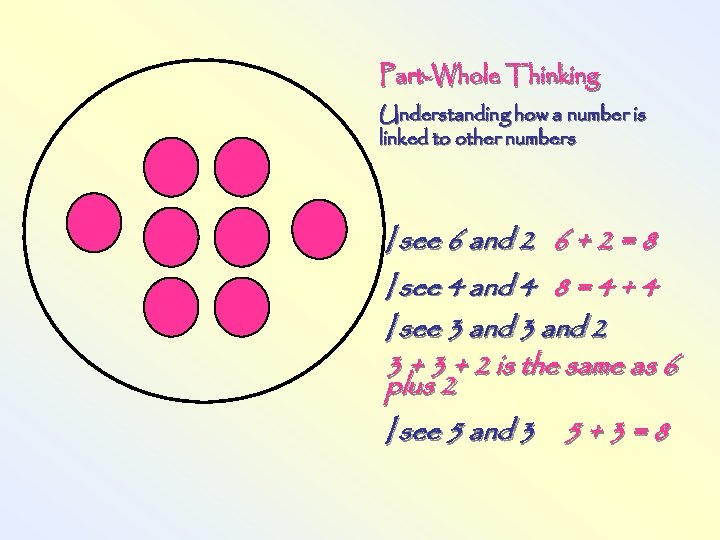Part-Whole Thinking Understanding how a number is linked to other numbers I see 6 and 2 6 + 2 = 8 I see 4 and 4 8 = 4 + 4 I see 3 and 2 3 + 2 is the same as 6 plus 2 I see 5 and 3 5 + 3 = 8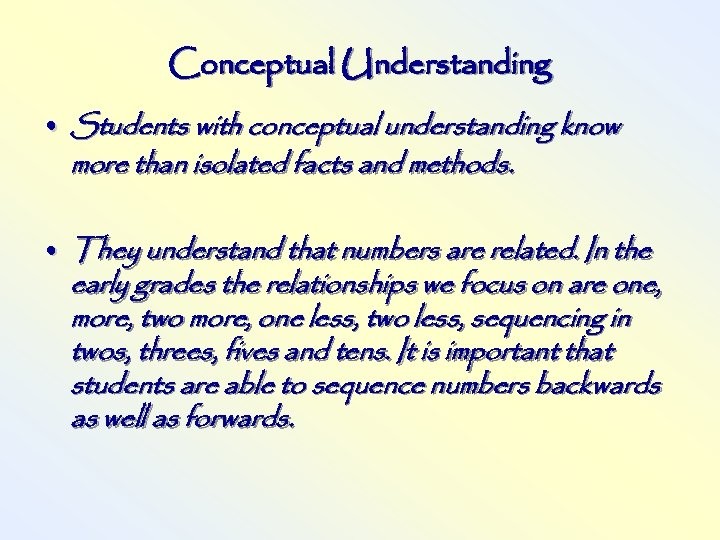Conceptual Understanding • Students with conceptual understanding know more than isolated facts and methods. • They understand that numbers are related. In the early grades the relationships we focus on are one, more, two more, one less, two less, sequencing in twos, threes, fives and tens. It is important that students are able to sequence numbers backwards as well as forwards.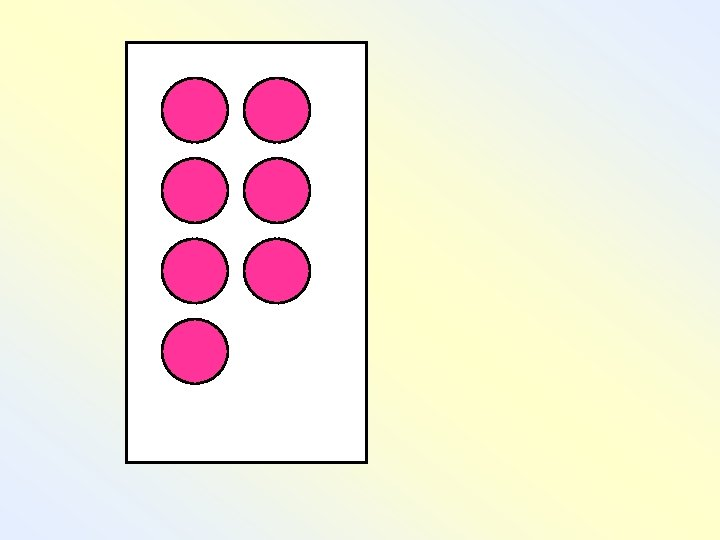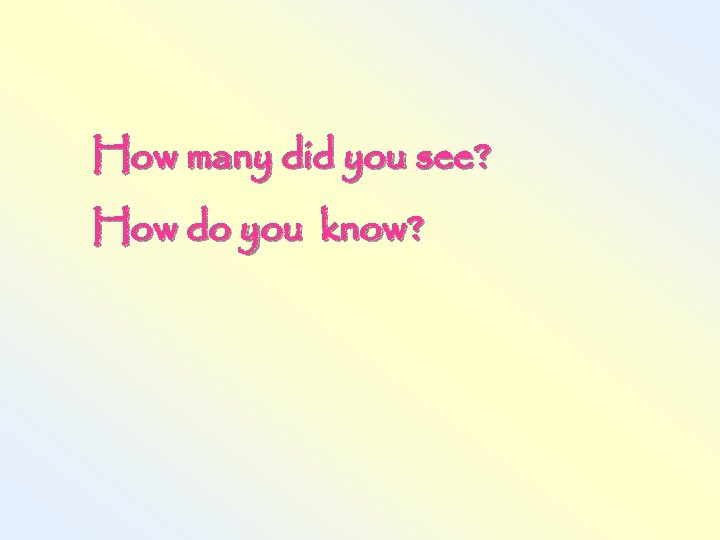How many did you see? How do you know?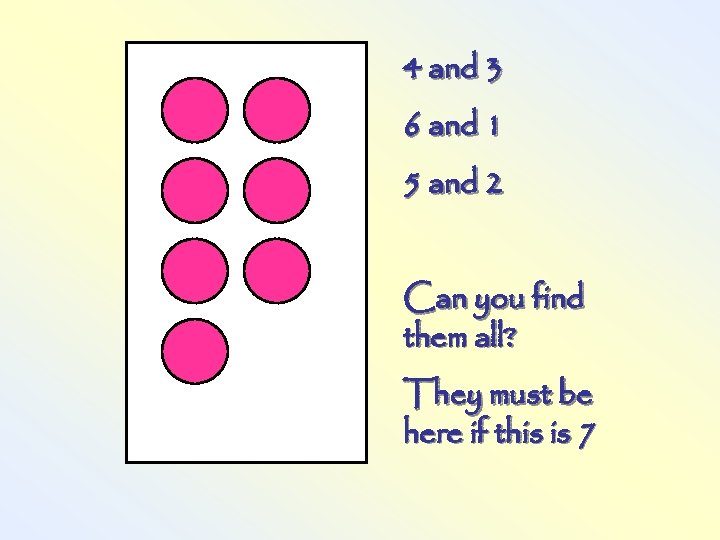4 and 3 6 and 1 5 and 2 Can you find them all? They must be here if this is 7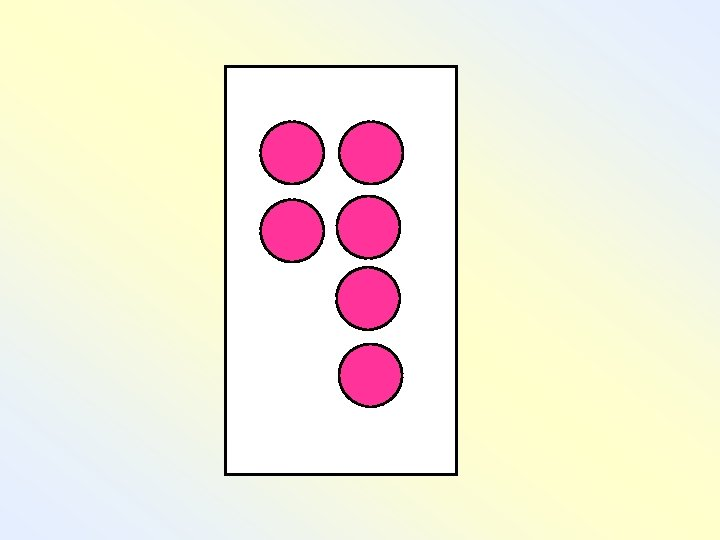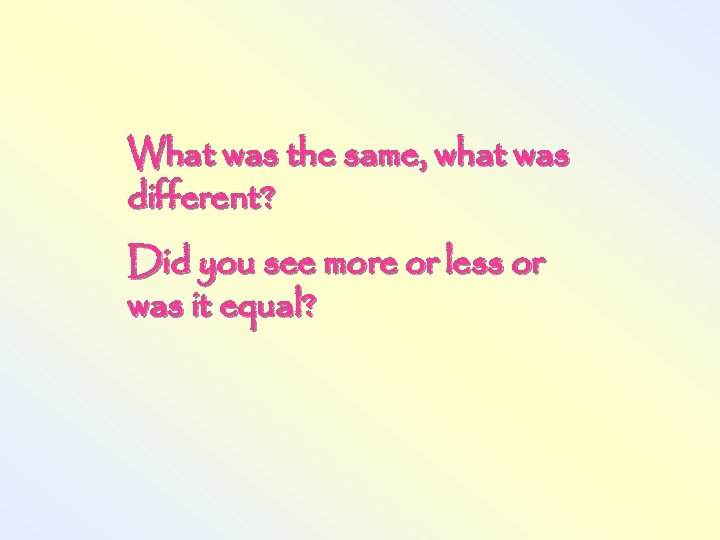What was the same, what was different? Did you see more or less or was it equal?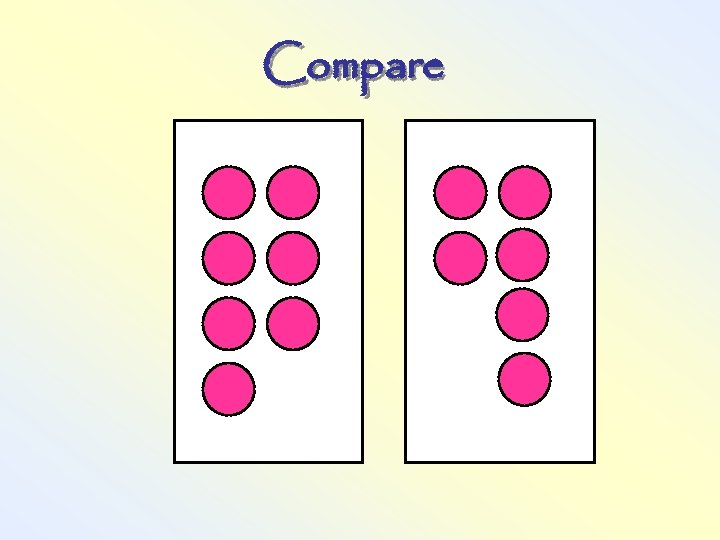Compare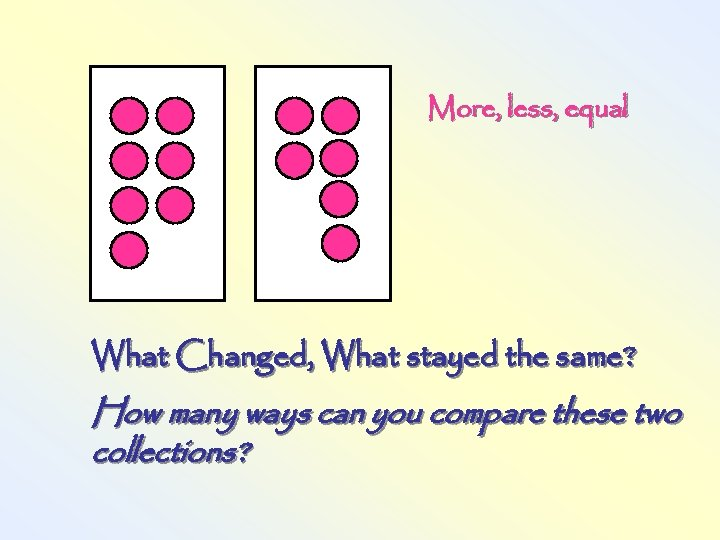More, less, equal What Changed, What stayed the same? How many ways can you compare these two collections?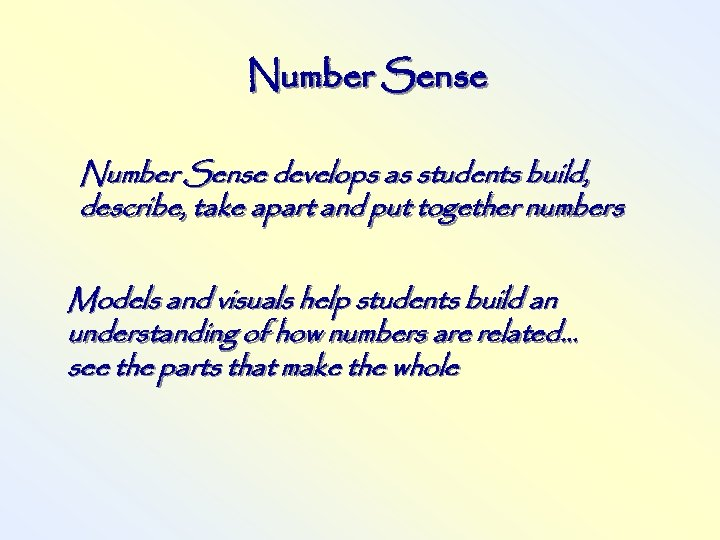Number Sense develops as students build, describe, take apart and put together numbers Models and visuals help students build an understanding of how numbers are related… see the parts that make the whole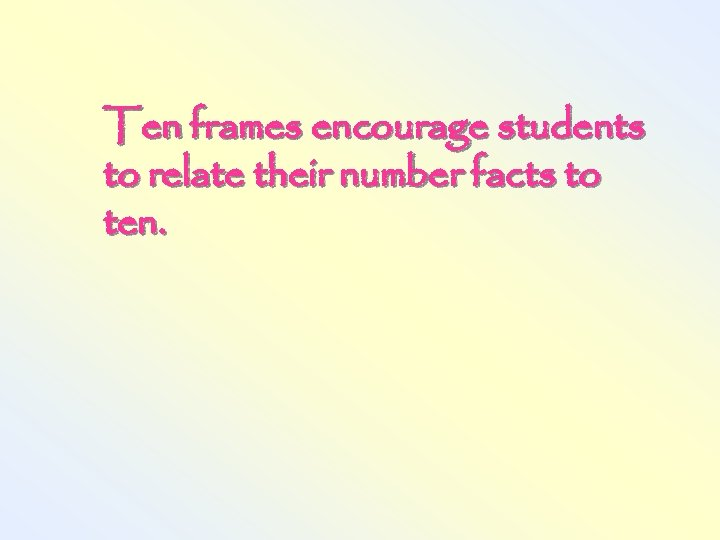Ten frames encourage students to relate their number facts to ten.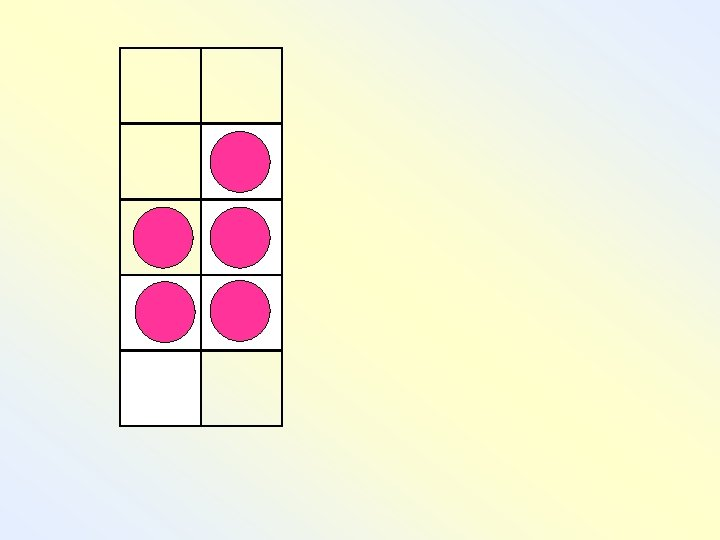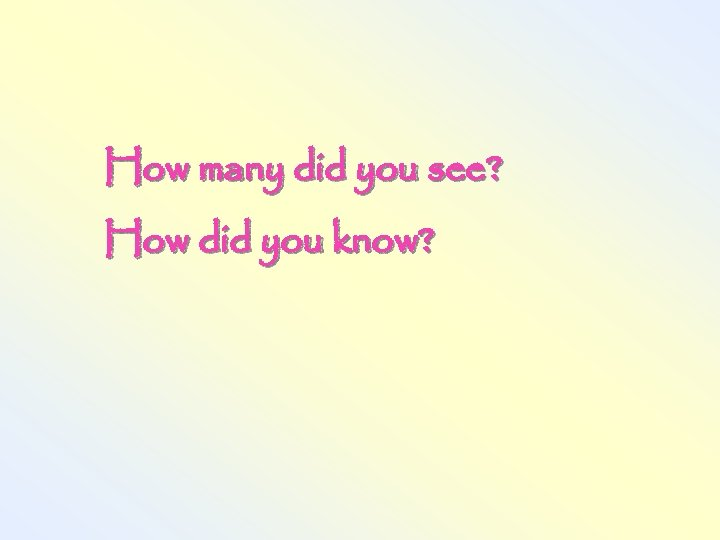How many did you see? How did you know?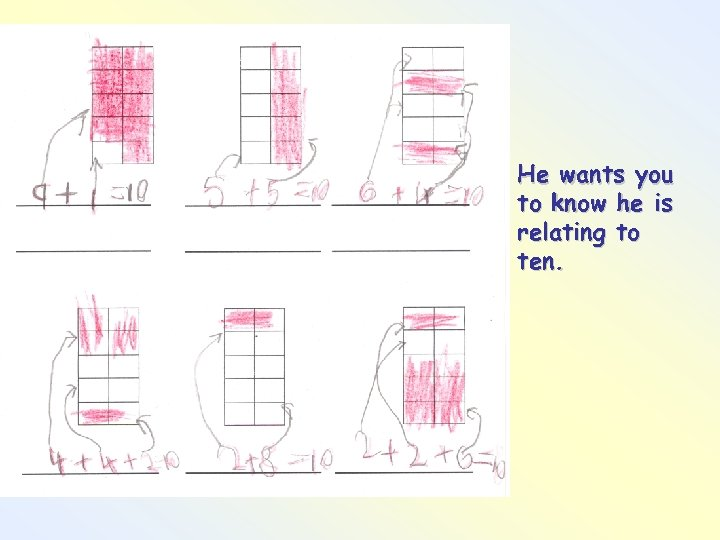He wants you to know he is relating to ten.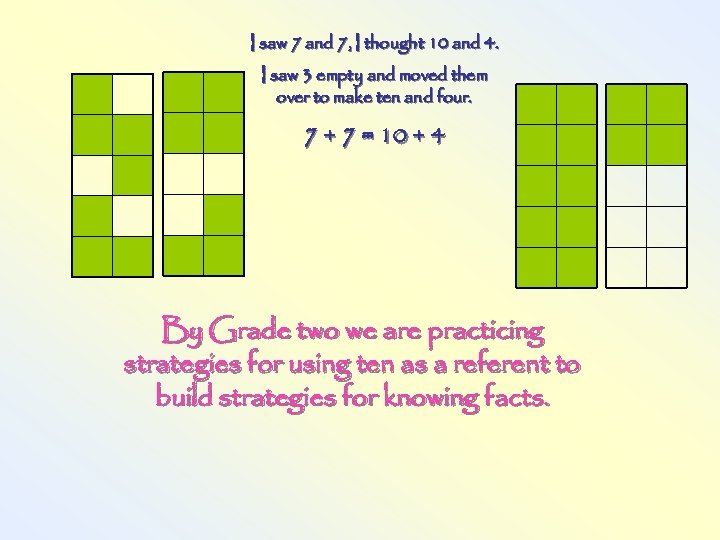I saw 7 and 7, I thought 10 and 4. I saw 3 empty and moved them over to make ten and four. 7 + 7 = 10 + 4 By Grade two we are practicing strategies for using ten as a referent to build strategies for knowing facts.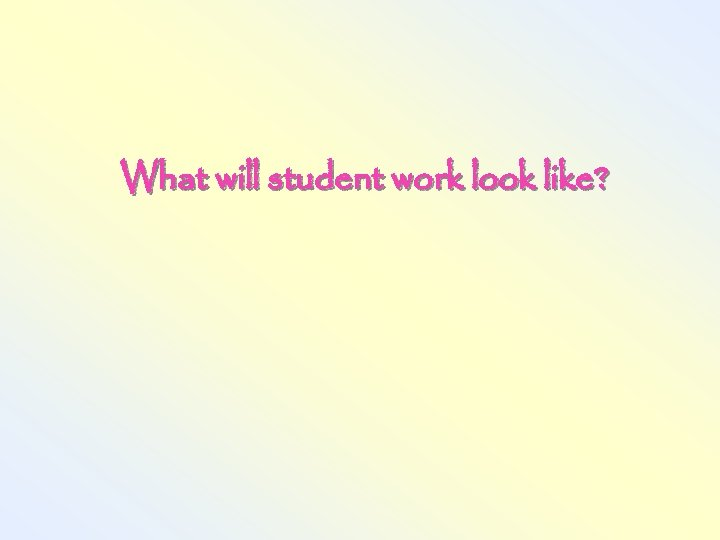What will student work look like?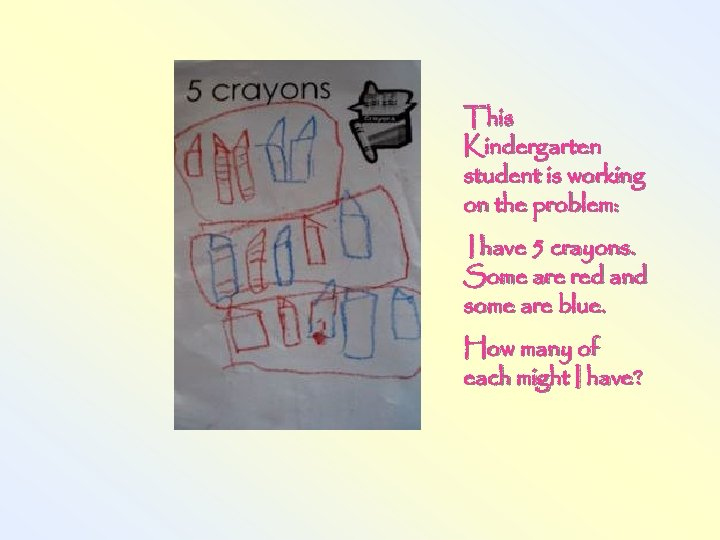This Kindergarten student is working on the problem: I have 5 crayons. Some are red and some are blue. How many of each might I have?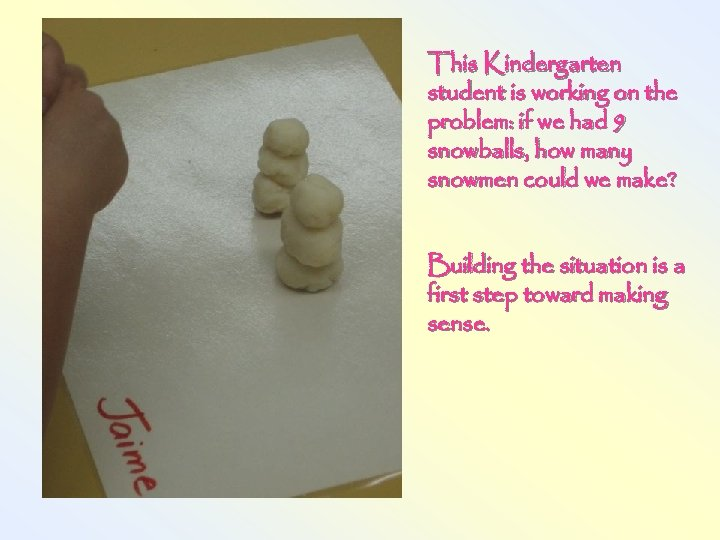This Kindergarten student is working on the problem: if we had 9 snowballs, how many snowmen could we make? Building the situation is a first step toward making sense.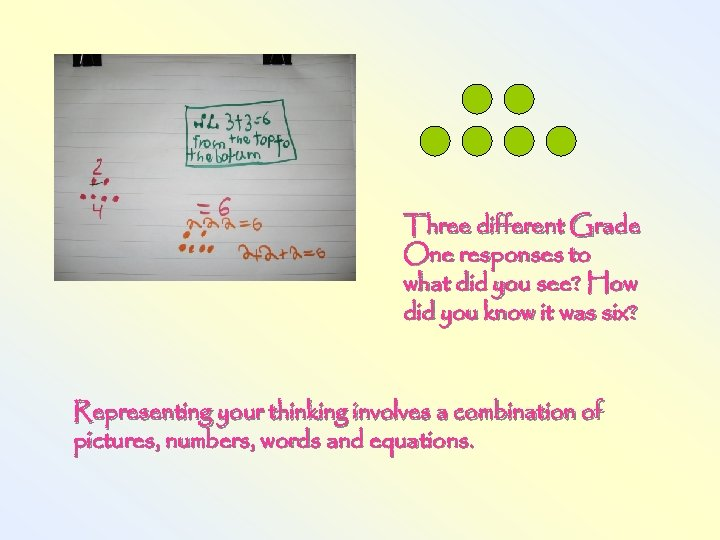Three different Grade One responses to what did you see? How did you know it was six? Representing your thinking involves a combination of pictures, numbers, words and equations.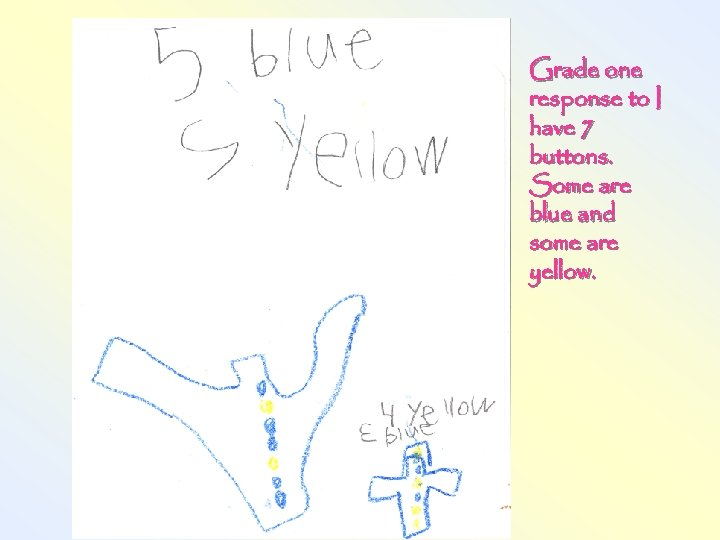Grade one response to I have 7 buttons. Some are blue and some are yellow.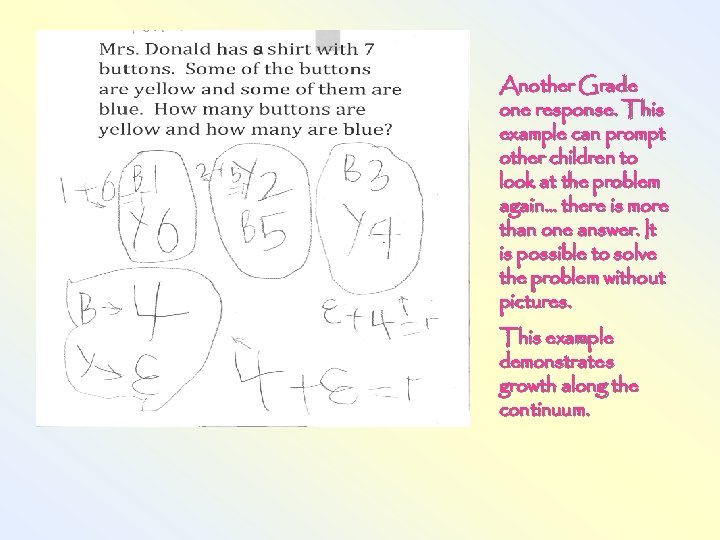Another Grade one response. This example can prompt other children to look at the problem again… there is more than one answer. It is possible to solve the problem without pictures. This example demonstrates growth along the continuum.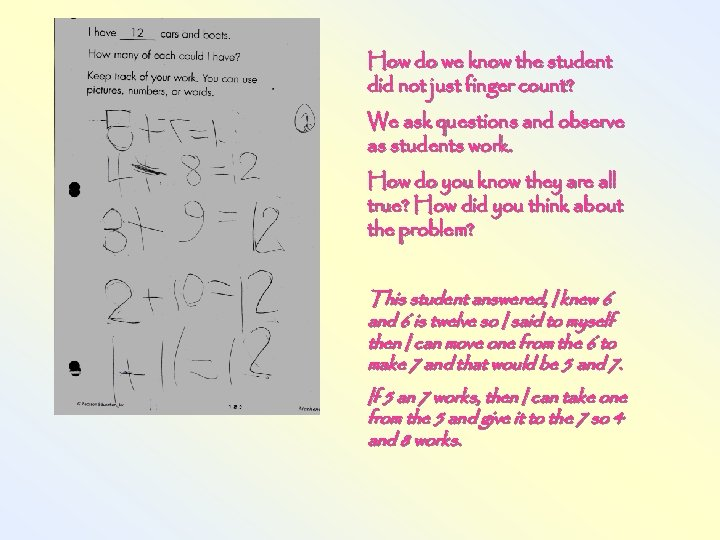How do we know the student did not just finger count? We ask questions and observe as students work. How do you know they are all true? How did you think about the problem? This student answered, I knew 6 and 6 is twelve so I said to myself then I can move one from the 6 to make 7 and that would be 5 and 7. If 5 an 7 works, then I can take one from the 5 and give it to the 7 so 4 and 8 works.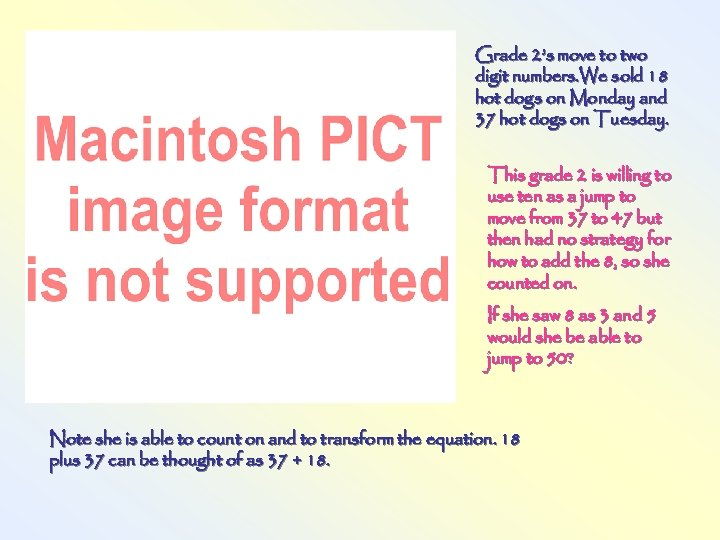Grade 2’s move to two digit numbers. We sold 18 hot dogs on Monday and 37 hot dogs on Tuesday. This grade 2 is willing to use ten as a jump to move from 37 to 47 but then had no strategy for how to add the 8, so she counted on. If she saw 8 as 3 and 5 would she be able to jump to 50? Note she is able to count on and to transform the equation. 18 plus 37 can be thought of as 37 + 18.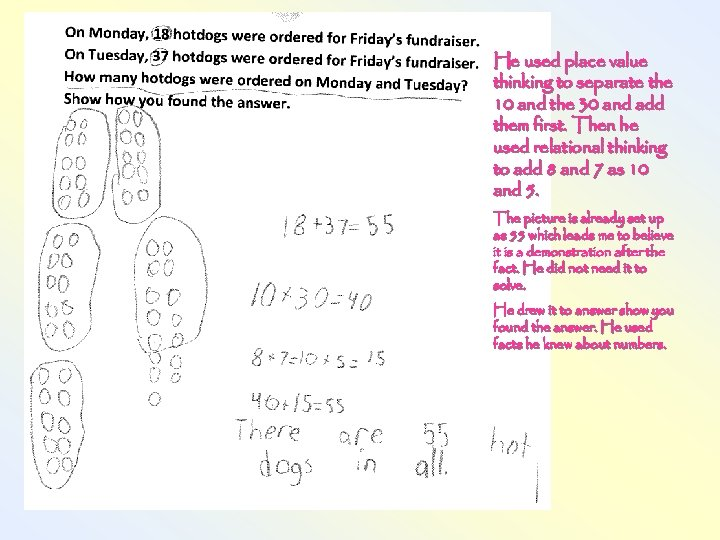He used place value thinking to separate the 10 and the 30 and add them first. Then he used relational thinking to add 8 and 7 as 10 and 5. The picture is already set up as 55 which leads me to believe it is a demonstration after the fact. He did not need it to solve. He drew it to answer show you found the answer. He used facts he knew about numbers.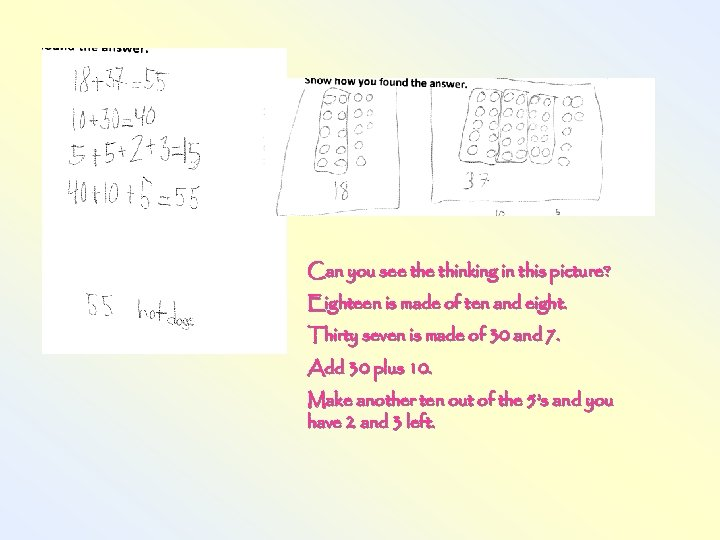Can you see thinking in this picture? Eighteen is made of ten and eight. Thirty seven is made of 30 and 7. Add 30 plus 10. Make another ten out of the 5’s and you have 2 and 3 left.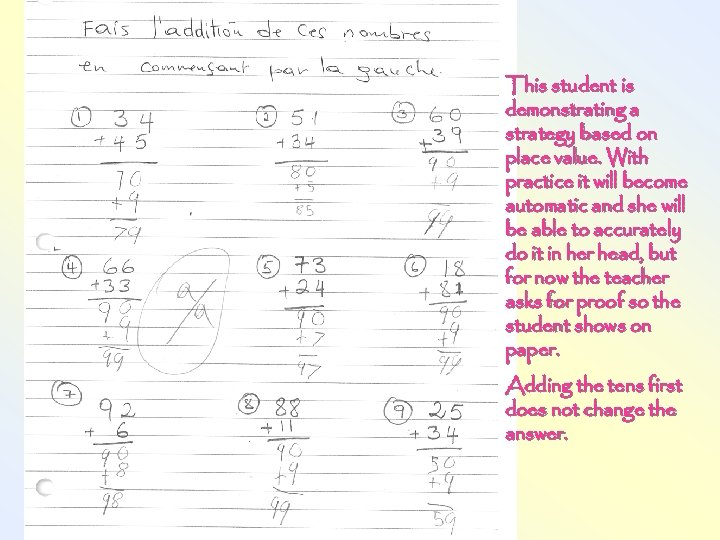This student is demonstrating a strategy based on place value. With practice it will become automatic and she will be able to accurately do it in her head, but for now the teacher asks for proof so the student shows on paper. Adding the tens first does not change the answer.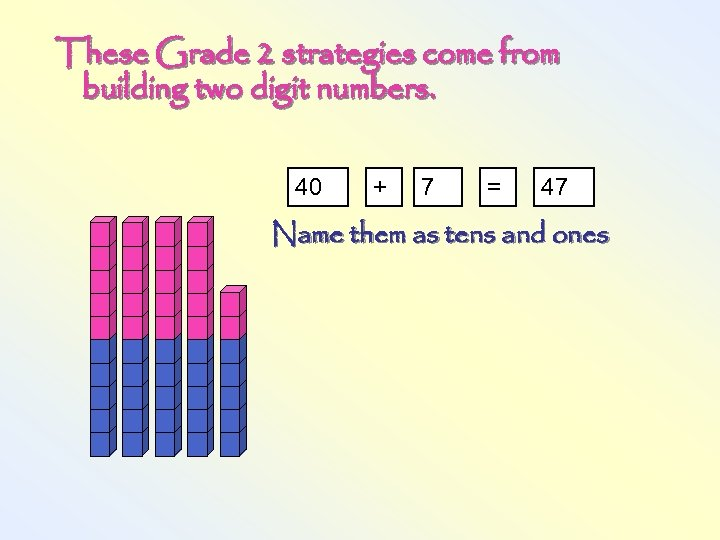These Grade 2 strategies come from building two digit numbers. 40 + 7 = 47 Name them as tens and ones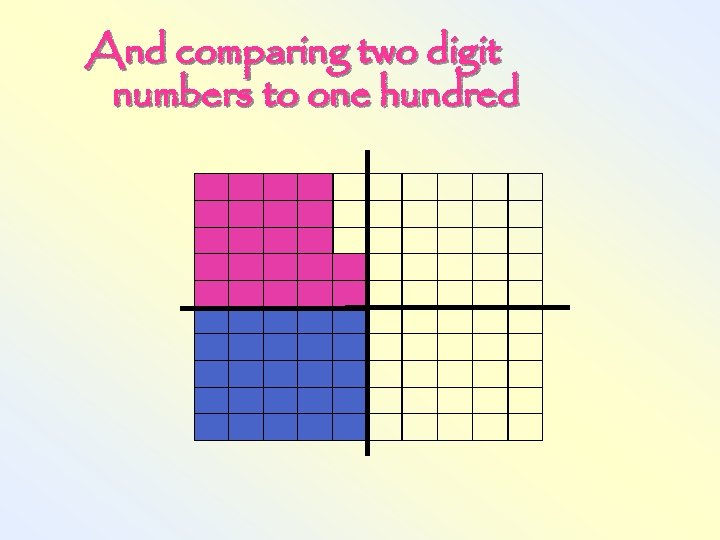And comparing two digit numbers to one hundred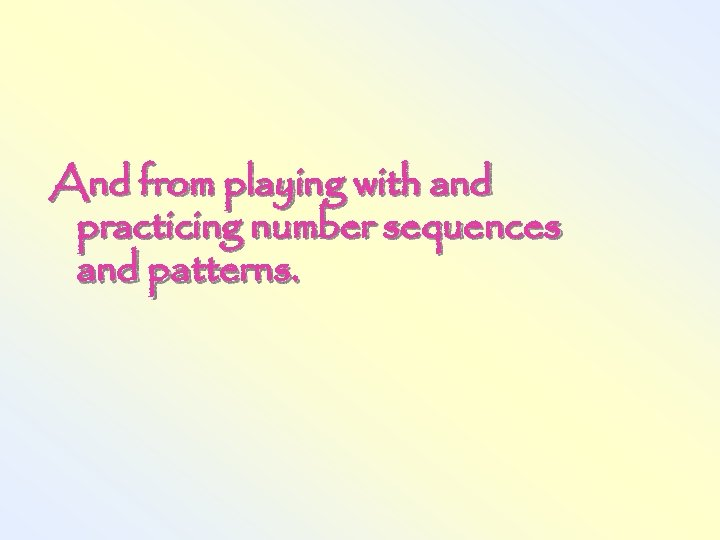And from playing with and practicing number sequences and patterns.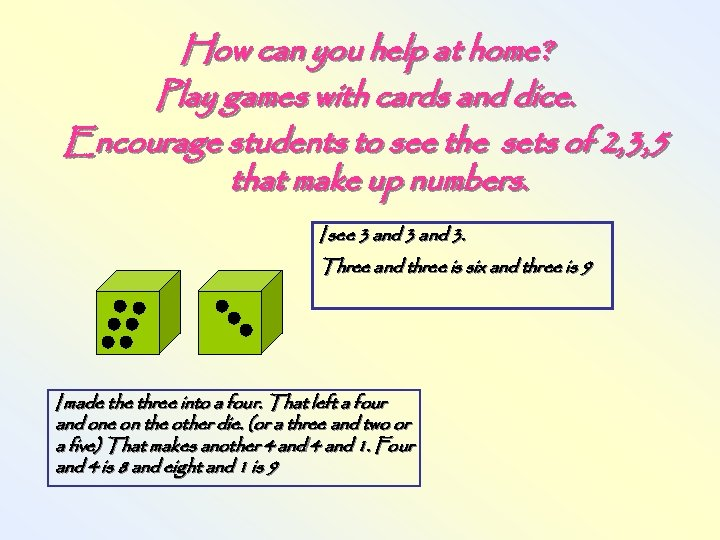How can you help at home? Play games with cards and dice. Encourage students to see the sets of 2, 3, 5 that make up numbers. I see 3 and 3. Three and three is six and three is 9 I made three into a four. That left a four and one on the other die. (or a three and two or a five) That makes another 4 and 1. Four and 4 is 8 and eight and 1 is 9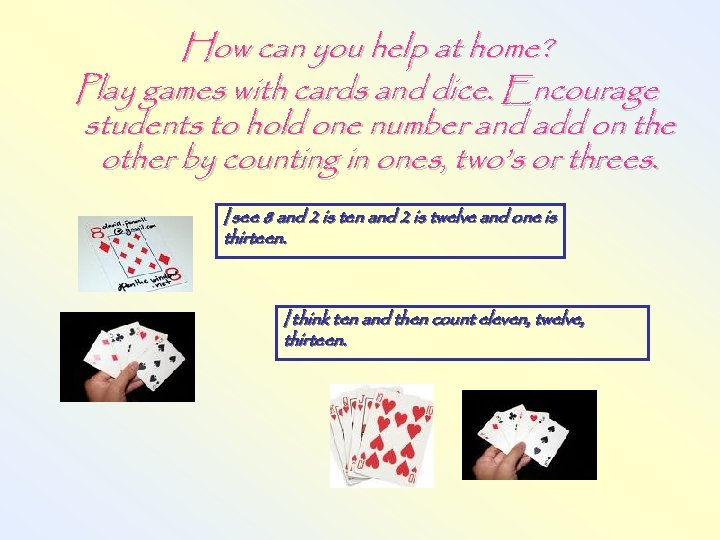How can you help at home? Play games with cards and dice. Encourage students to hold one number and add on the other by counting in ones, two’s or threes. I see 8 and 2 is ten and 2 is twelve and one is thirteen. I think ten and then count eleven, twelve, thirteen.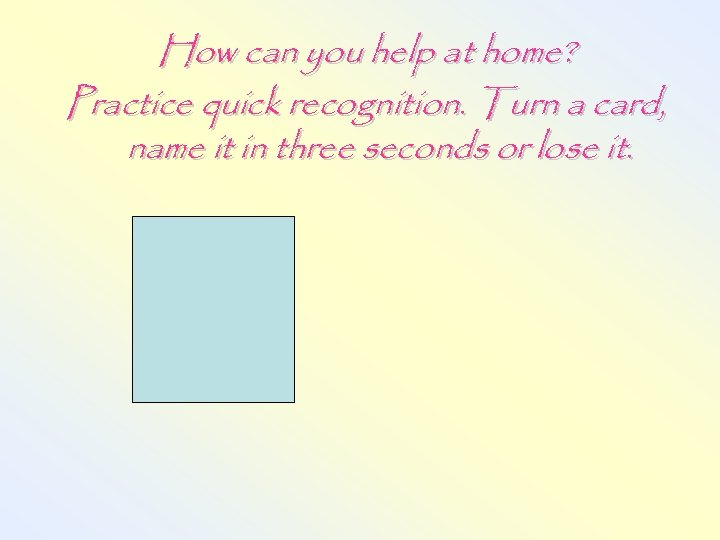How can you help at home? Practice quick recognition. Turn a card, name it in three seconds or lose it.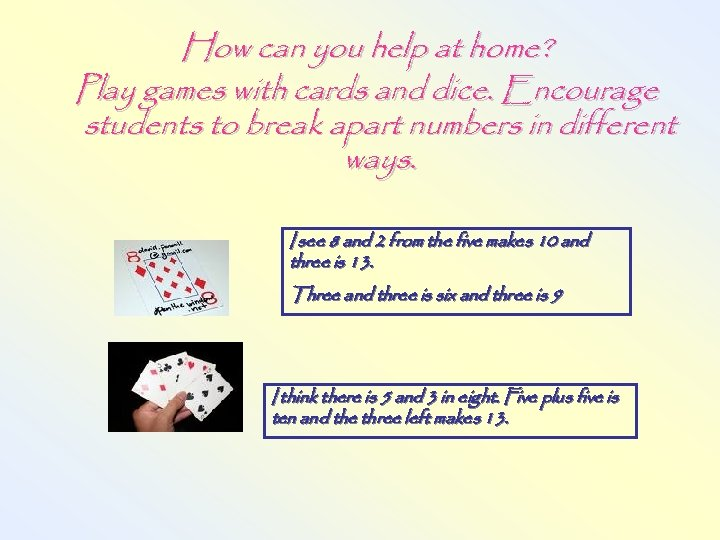How can you help at home? Play games with cards and dice. Encourage students to break apart numbers in different ways. I see 8 and 2 from the five makes 10 and three is 13. Three and three is six and three is 9 I think there is 5 and 3 in eight. Five plus five is ten and the three left makes 13.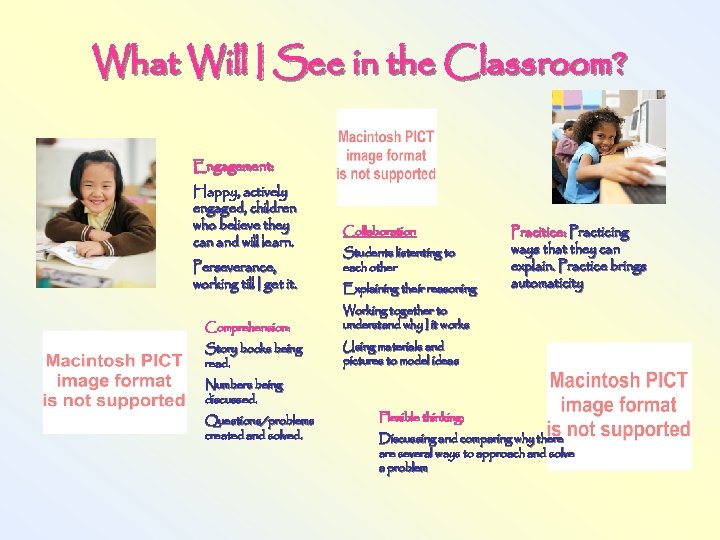What Will I See in the Classroom? Engagement: Happy, actively engaged, children who believe they can and will learn. Perseverance, working till I get it. Comprehension: Story books being read. Numbers being discussed. Questions/problems created and solved. Collaboration Students listenting to each other Explaining their reasoning Pracitice: Practicing ways that they can explain. Practice brings automaticity Working together to understand why I it works Using materials and pictures to model ideas Flexible thinking: Discussing and comparing why there are several ways to approach and solve a problem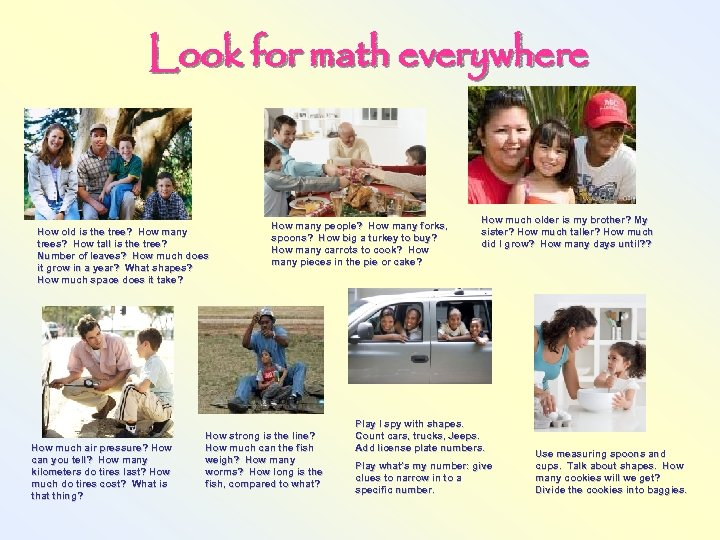Look for math everywhere How old is the tree? How many trees? How tall is the tree? Number of leaves? How much does it grow in a year? What shapes? How much space does it take? How much air pressure? How can you tell? How many kilometers do tires last? How much do tires cost? What is that thing? How many people? How many forks, spoons? How big a turkey to buy? How many carrots to cook? How many pieces in the pie or cake? How strong is the line? How much can the fish weigh? How many worms? How long is the fish, compared to what? How much older is my brother? My sister? How much taller? How much did I grow? How many days until? ? Play I spy with shapes. Count cars, trucks, Jeeps. Add license plate numbers. Play what’s my number: give clues to narrow in to a specific number. Use measuring spoons and cups. Talk about shapes. How many cookies will we get? Divide the cookies into baggies.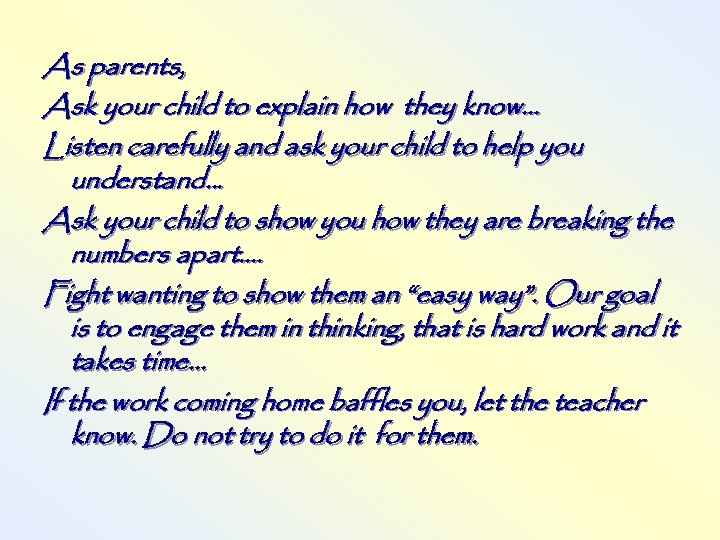As parents, Ask your child to explain how they know. . . Listen carefully and ask your child to help you understand. . . Ask your child to show you how they are breaking the numbers apart. . Fight wanting to show them an “easy way”. Our goal is to engage them in thinking, that is hard work and it takes time. . . If the work coming home baffles you, let the teacher know. Do not try to do it for them.